Announcements

# Missing Manual - ValueLoop() & ValueList()

cancel
Showing results for
Did you mean:Master III

## Missing Manual - ValueLoop() & ValueList()Have you ever wondered how the examples from the Qlikview help may look like?

Please see below and enjoy responsibly...

Valueloop() & VaueList()

Both of those functions belong to Synthetic Dimension Functions.

Synthetic Dimension is a type of Calculated Dimension

- the difference between "standard" Calculated Dimension and Synthetic one is that

the standard dimensions are based on values from existing fields

whereas for Synthetic Dimensions those values are created "on the fly".

The drawback here is that you can not mix standard dimensions with synthetic in the way you would expect.

ValueLoop()

Used:

Back End -No

Front End - YesDescription(Qlikview Help)

Returns a set of iterated values which, when used in a calculated dimension, will form a synthetic dimension.

The values generated will start with the from value and end with the to value including intermediate values in increments of step. In charts with a synthetic dimension created with the valueloop function it is possible to reference the dimension value corresponding to a specific expression cell by restating the valuloop function with the same parameters in the chart expression. The function may of course be used anywhere in the layout, but apart from when used for synthetic dimensions it will only be meaningful inside an aggregation functionCreate series of numbers in a range given by criteria.from - first value

to - last value

step - intermediate values criteria.

When step is missing 1 is assumed

Qlikview help examples are very straightforwards and easy to understand:

Example 1Example 2Example 3

valueloop ( 1, 3 )

From 1 to 3, (step is omitted so 1 is assumed):

valueloop ( 1, 5, 2 )

From 1 to 5, step 2:

1,1+2=3,3+2=5

valueloop ( 11 )

returns the value 11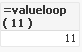Practical use

Example 1

If you need to provide calculations to check if the MOD of values from 0 to 100 with step 5 is divided by 10 without remainder

Create Dimension: ValueLoop(0,100,5)

and Expression: if(mod(ValueLoop(0,100,5),10)=0,'OK', 'No OK')How to create a Square Pie Chart

or

qlikfreak.wordpress.com/2014/06/17/infographics-in-qlikview-vol-2/

ValueList()

Used:

Back End -No

Front End - YesDescription(Qlikview Help)

Returns a set of listed values which, when used in a calculated dimension,

will form a synthetic dimension. In charts with a synthetic dimension created with the valuelist function it is possible to reference the dimension value corresponding to a specific expression cell by restating the valuelist function with the same parameters in the chart expression. The function may of course be used anywhere in the layout, but apart from when used for synthetic dimensions it will only be meaningful inside an aggregation functionCreate series of values from given listv1 - list of values

Again, both of those examples are very easy to understand:

valuelist ( 1, 10, 100 )valuelist ( 'a', 'xyz', 55 )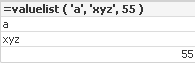Practical use

Data Model

Year(Date) as Year,

Values

inline [

Date, Values

01/01/2009, 1

11/04/2009, 2

20/07/2009, 2

28/10/2009, 2

05/02/2010, 2

16/05/2010, 2

24/08/2010, 1

02/12/2010, 1

]

The usual way of creating straight table is be to add Year as Dimension and sum(Values) as expression.

This will return value for each Year.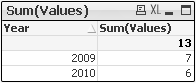But if we want o use Synthetic Dimension in the same way this will return only one Total value for both years.One of the way to use this function is to create list (similar to Statistics Box) with your own KPI's: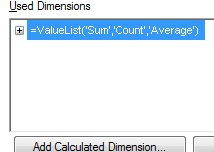and then use nested IF statement to create your Metrics=if(ValueList('Sum','Count','Average')='Sum',Sum(Values),

if(ValueList('Sum','Count','Average')='Count',Count(Values),Avg(Values)))

to get below result:As nesting IF's can be tricky and cumbersome beyond 2 or 3 criteria we can use Pick/Match functions to improve our calculations:

=pick(match(ValueList('Sum','Count','Average'),'Sum','Count','Average')

,Sum(Values),Count(Values),Avg(Values))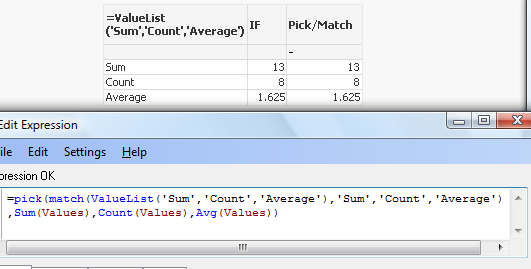Conclusion:

Although both of those function are not very often used

(they did not make to final 30 of  rwunderlich survey

Help with QV Function Survey‌

you can find a practical way of using them.

Feeling Qlikngry?

How To /Missing Manual(19 articles)

Labels (5)

• ### QlikView ApplicationsCreator III

Thanks for sharing! Interesting article, finally clarifying some of my questions regarding ValueList()!Creator III

I used this in my dimension to get a list of all the dimensions

 =ValueList( \$(=Concat(chr(39)& \$Field & chr(39) , ', ' )) )

Would it be possible to make a second dimension with the values of these dimensions?

Then it would be possible to count the number of records you have for each value. This opens possibilities to compare groups in different states.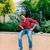MVP

Nice post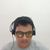Specialist

Very good.Not applicable

Muy buen aporte.

Por fin he entendido ValueList() y ValueLoop()Master III